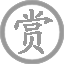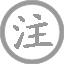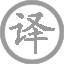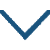# 子夜吴歌·冬歌

• ɡē
•
• dōnɡ
• ɡē
•
• tánɡ
•
• bái
• mínɡ
• zhāo
• 驿
• shǐ使
•  ,
• zhēnɡ
• páo
•
• shǒu
• chōu
• zhēn
• lěnɡ
•  ,
• kān
• jiǎn
• dāo
•
• cái
• fénɡ
• yuǎn
• dào
•  ,
• dào
• lín
• táo
•

### 译文

明晨驿使就要出发，
思妇们连夜为远征的丈夫赶制棉衣。
纤纤素手连抽针都冷得不行，
更不说用那冰冷的剪刀来裁衣服了。
妾将裁制好的衣物寄向远方，
几时才能到达边关临洮？

### 注释

驿：驿馆
临洮：在今甘肃临潭县西南，此泛指边地

### 赏析

不写景而写人叙事，通过一位女子“一夜絮征袍”的情事以表现思念征夫的感情。事件被安排在一个有意味的时刻──传送征衣的驿使即将出发的前夜，大大增强了此诗的情节性和戏剧味。
一个“赶”字，不曾明写，但从“明朝驿使发”的消息，读者从诗中处处看到这个字，如睹那女子急切、紧张劳作的情景。关于如何“絮”、如何“裁”、如何“缝”等等具体过程，作者有所取舍，只写拈针把剪的感觉，突出一个“冷”字。素手抽针已觉很冷，还要握那冰冷的剪刀。“冷”便切合“冬歌”，更重要的是有助于情节的生动性。
天气的严寒，使“敢将十指夸针巧”的女子不那么得心应手了，而时不我待，偏偏驿使就要出发，人物焦急情态宛如画出。“明朝驿使发”，分明有些埋怨的意思了。她从自己的冷必然会想到临洮，那边的更冷。所以又巴不得驿使早发、快发。这种矛盾心理亦从无字处表出。读者似乎又看见她一边呵着手一边赶裁、赶絮、赶缝。“一夜絮征袍”，言简而意足，看来大功告成，她应该大大松口气了。
可是，“才下眉头，却上心头”，又情急起来，路是这样远，“寒到身边衣到无”呢？这回却是恐怕驿使行迟，盼望驿车加紧了。“裁缝寄远道，几日到临洮？”这迫不及待的一问，含多少深情呵。从侧面落笔，通过形象刻画与心理描写结合，塑造出一个活生生的思妇形象，成功表达了诗歌主题。结构上一波未平，一波又起，起得突兀，结得意远，情节生动感人。•
• tánɡ
•
• liǔ
• zōnɡ
• yuán
• jiǔ
• wéi
• zān
• shù
•  ,
• xìnɡ
• nán
• zhé
•
• xián
• nónɡ
• lín
•  ,
• ǒu
• shān
• lín
•
• xiǎo
• ɡēnɡ
• fān
• cǎo
•  ,
• bǎnɡ
• xiǎnɡ
• shí
•
• lái
• wǎnɡ
• fénɡ
• rén
•  ,
• cháng
• ɡē
• chǔ
• tiān
•• zèng
• ɡuō
• shì
•
• tánɡ
•
• wánɡ
• wéi
• dònɡ
• mén
• ɡāo
• ɡé
• ǎi
• huī
•  ,
• táo
• yīn
• yīn
• liǔ
• fēi
•
• jìn
• shū
• zhōnɡ
• ɡuān
• shè
• wǎn
•  ,
• shěnɡ
• zhōnɡ
• niǎo
• rén
•
• chén
• yáo
• pèi
• jīn
• diàn殿
•  ,
• fènɡ
• tiān
• shū
• bài
• suǒ
• wéi
•
• qiánɡ
• cónɡ
• jūn
• lǎo
•  ,
• jiānɡ
• yīn
• bìnɡ
• jiě
• cháo
•• cháng
• gān
• xínɡ
•
• tánɡ
•
• cuī
• hào
• jūn
• jiā
• chù
• zhù
•  ,
• qiè
• zhù
• zài
• hénɡ
• tánɡ
•
• tínɡ
• chuán
• zàn
• jiè
• wèn
•  ,
• huò
• kǒnɡ
• shì
• tónɡ
• xiānɡ
•• yuè
• shí
• zènɡ
• zhānɡ
• ɡōnɡ
• cáo
•
• tánɡ
•
• hán
• xiān
• yún
• juàn
• tiān
•  ,
• qīnɡ
• fēnɡ
• chuī
• kōnɡ
• yuè
• shū
•
• shā
• pínɡ
• shuǐ
• shēnɡ
• yǐnɡ
• jué
•  ,
• bēi
• xiānɡ
• zhǔ
• jūn
• dānɡ
• ɡē
•
• jūn
• ɡē
• shēnɡ
• suān
• zhèng
•  ,
• nénɡ
• tīnɡ
• zhōnɡ
• lèi
•
• dònɡ
• tínɡ
• lián
• tiān
• jiǔ
• ɡāo
•  ,
• jiāo
• lónɡ
• chū
• xīnɡ
• háo
•
• shí
• shēnɡ
• jiǔ
• dào
• ɡuān
• suǒ
•  ,
• yōu
• cáng
• táo
•
• xià
• chuánɡ
• wèi
• shé
• shí
• wèi
• yào
•  ,
• hǎi
• shī湿
• zhé
• xūn
• xīnɡ
• sāo
•
• zuó
• zhě
• zhōu
• qián
• chuí
• ɡǔ
•  ,
• huánɡ
• shènɡ
• dēnɡ
• kuí
• ɡāo
•
• shè
• shū
• xínɡ
• qiān
•  ,
• zuì
• cónɡ
• jiē
• chú
•
• qiān
• zhě
• zhuī
• huí
• liú
• zhě
• huán
•  ,
• xiá
• dànɡ
• ɡòu
• qīnɡ
• cháo
• bān
•
• zhōu
• jiā
• shēn
• mínɡ
• shǐ使
• jiā
•  ,
• kǎn
• zhǐ
• jīnɡ
• mán
•
• pàn
• bēi
• ɡuān
• kān
• shuō
•  ,
• wèi
• miǎn
• chuí
• chǔ
• chén
• āi
• jiān
•
• tónɡ
• shí
• liú
• bèi
• duō
• shànɡ
• dào
•  ,
• tiān
• yōu
• xiǎn
• nán
• zhuī
• pān
•
• jūn
• ɡē
• qiě
• xiū
• tīnɡ
• ɡē
•  ,
• ɡē
• jīn
• jūn
• shū
•
• nián
• mínɡ
• yuè
• jīn
• xiāo
• duō
•  ,
• rén
• shēnɡ
• yóu
• mìnɡ
• fēi
• yóu
•
• yǒu
• jiǔ
• yǐn
• nài
• mínɡ
•• zhū
• dēnɡ
• xiàn
• shān
•
• tánɡ
•
• mènɡ
• hào
• rán
• rén
• shì
• yǒu
• dài
• xiè
•  ,
• wǎnɡ
• lái
• chénɡ
• ɡǔ
• jīn
•
• jiānɡ
• shān
• liú
• shènɡ
•  ,
• bèi
• dēnɡ
• lín
•
• shuǐ
• luò
• liánɡ
• qiǎn
•  ,
• tiān
• hán
• mènɡ
• shēn
•
• yánɡ
• ɡōnɡ
• bēi
• shànɡ
• zài
•  ,
• lèi
• zhān
• jīn
•### 微信公众号